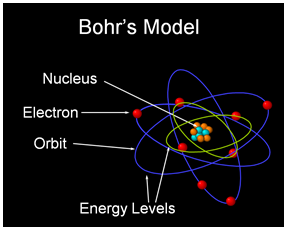# Niels Bohr Atomic Model And Limitations## Bohr’s Theory – Bohr’s Atomic ModelNiels Bohr atomic model

Thomson’s atomic model and Rutherford’s atomic model failed to answer any questions related to the energy of an atom and its stability. In the year 1913, Niels Bohr proposed an atomic structure model, describing an atom as a small, positively charged nucleus surrounded by electrons that travel in circular orbits around the positively charged nucleus as planets around the sun in our solar system, with attraction provided by electrostatic forces, popularly known as Bohr’s atomic model. It was basically an improved version of Rutherford’s atomic model overcoming its limitations. On most of the points, he is in agreement with him, like concepts of nucleus and electrons orbiting it. Salient features of Niels Bohr atomic model are:

• Electrons revolve around the nucleus in stable orbits without emission of radiant energy. Each orbit has a definite energy and is called an energy shell or energy level.
• An orbit or energy level is designated as K, L, M, N shells. When the electron is in the lowest energy level, it is said to be in the ground state.
• An electron emits or absorbs energy when it jumps from one orbit or energy level to another. When it jumps from a higher energy level to lower energy level it emits energy while it absorbs energy when it jumps from a lower energy level to a higher energy level.
• The energy absorbed or emitted is equal to the difference between the energies of the two energy levels (E1, E2) and is determined by Plank’s equation.

ΔE = E2-E1 = hv

Where,

ΔE = energy absorbed or emitted
h= Plank’s constant
v= frequency of electromagnetic radiation emitted or absorbed

• The angular momentum of an electron revolving in energy shells is given by:

mevr = nh/2π

Where,
n= number of corresponding energy shell; 1, 2, 3 …..
me= mass of the electron
v= velocity